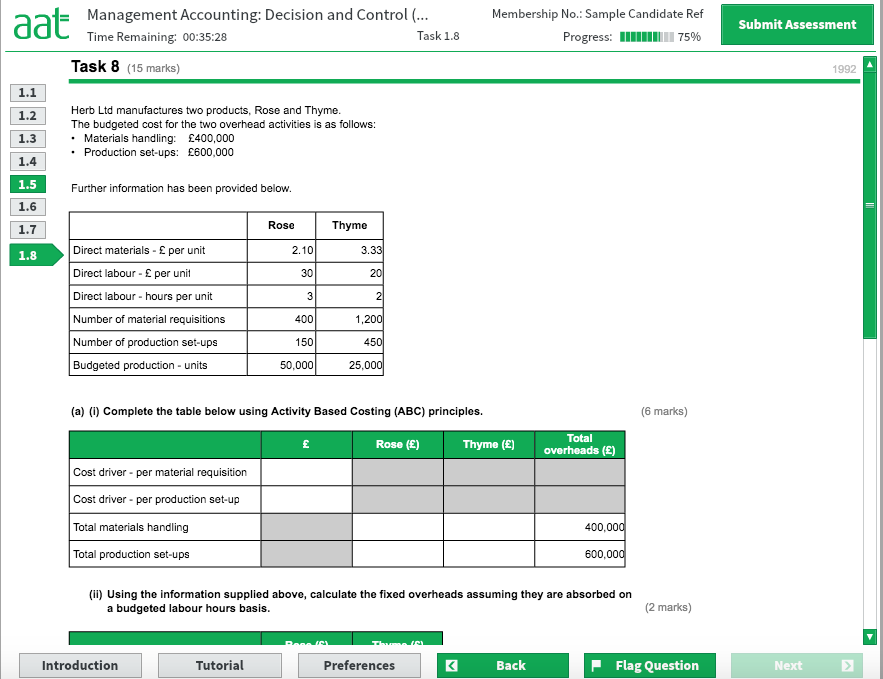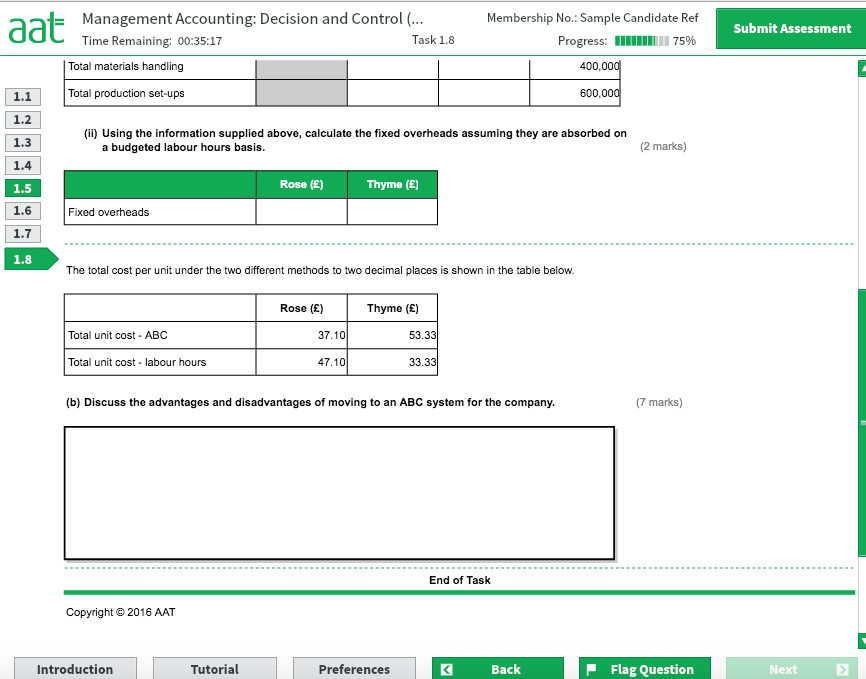# Task 8 Moc2

Hi Everyone,

I was hoping someone could help me get my head around this task please?

Thanks so much in advance x## Comments

• Hi

The task is testing ABC costing and comparing it to labour hours costing.

In a) i) you're calculating the total allocated overheads based on the cost drivers provided. In ii) you're calculating the total overheads for each product on a labour hours basis.

For b) you're looking at the differences between the methods and how they represent the costs. I won't go through this in the post.

a)
The best way to approach this is look at what you need before doing too much calculation.

You need the total number of: material requisitions, production set ups and labour hours. If the question was more complex you might also want to jot down the total fixed cost.

Material requisitions = 400+1200 = 1,600
Set ups = 150+450 = 600
Labour hours = 3*50000+2*25000 = 200,000
Total Fixed cost = 600000+400000=1,000,000

Now you have all the pieces to answer the question fully.

i)
Cost per material requisition = 400000/1600 = 250
Cost per production set up = 600000/600 = 1,000

Material Handling = Cost per requisition * number of requisitions.
Rose = 400 * 250 = 100,000
Thyme = 1200 * 250 = 300,000

Production set up costs = Cost per set up * number of set ups
Rose = 150 * 1000 = 150,000
Thyme = 450 * 1000 = 450,000

ii)
Cost per labour hour = 1000000/200000 = £5

Total costs by labour hour:
Rose = 5*3*50000 = 750,000
Thyme = 5*2*25000 = 250,000

You are given a table which can help you check your results as well.

Total fixed cost for product/units + Direct labour + Direct material = unit cost

e.g Rose under ABC
250000/50000 + 30 + 2.1 =37.1 as given
AAT Level 4, MAAT
ACCA in progress
F4- Passed Aug 2020
F5- Passed Dec 2020
F6- Passed Sep 2020
F7- Planned June 2021
F8
F9 - Planned June 2021
Sign In or Register to comment.